# How to Calculate and Solve for Storage Co-efficient for a Confined Aquifer | Water Budget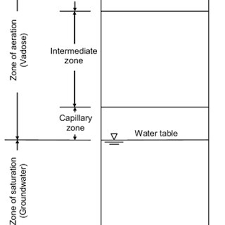The image above represents storage co-efficient for a confined aquifer.

To compute for storage co-efficient for a confined aquifer, five essential parameters are needed and these parameters are Unit Weight of Water (γw), Confined Aquifer Thickness (H), Compressibility of the Aquifer Material (α), Porosity of Aquifer Material (n) and Compressibility of Water (β).

The formula for calculating storage co-efficient for a confined aquifer:

S = γw(α + nβ)H

Where;

S = Storage Co-efficient for a Confined Aquifer
γw = Unit Weight of Water
H = Confined Aquifer Thickness
α = Compressibility of the Aquifer Material
n = Porosity of Aquifer Material
β = Compressibility of Water

Let’s solve an example;
Find the storage co-efficient for a confined aquifer when the unit weight of water is 8, confined aquifer thickness is 12, the compressibility of the aquifer material is 21, the porosity of aquifer material is 18 and the compressibiity of water is 14.

This implies that;

γw = Unit Weight of Water = 8
H = Confined Aquifer Thickness = 12
α = Compressibility of the Aquifer Material = 21
n = Porosity of Aquifer Material = 18
β = Compressibility of Water = 14

S = γw(α + nβ)H
S = 8(21 + 18(14))12
S = 8(21 + 252)12
S = 8(273)12
S = 26208

Therefore, the storage co-efficient for a confined aquifer is 26208.

Calculating the Unit Weight of Water when the Storage Co-efficient for a Confined Aquifer, the Confined Aquifer Thickness, the Compressibility of the Aquifer Material, the Porosity of Aquifer Material and the Compressibility of Water is Given.

γw = S / (a + nβ)H

Where;

γw = Unit Weight of Water
S = Storage Co-efficient for a Confined Aquifer
H = Confined Aquifer Thickness
α = Compressibility of the Aquifer Material
n = Porosity of Aquifer Material
β = Compressibility of Water

Let’s solve an example;
Find the unit weight of water when the storage co-efficient for a confined aquifer is 20, the confined aquifer thickness is 10, the compressibility of the aquifer material is 8, the porosity of aquifer material is 3 and the compressibility of water is 4.

This implies that;

S = Storage Co-efficient for a Confined Aquifer = 20
H = Confined Aquifer Thickness = 10
α = Compressibility of the Aquifer Material = 8
n = Porosity of Aquifer Material = 3
β = Compressibility of Water = 4

γw = S / (a + nβ)H
γw = 20 / (8 + (3 x 4))10
γw = 20 / (8 + 12)10
γw = 20 / (20)10
γw = 20 / 200
γw = 0.1

Therefore, the unit weight of water is 0.1.

Calculating the Confined Aquifer Thickness when the Storage Co-efficient for a Confined Aquifer, the Unit Weight of Water, the Compressibility of the Aquifer Material, the Porosity of Aquifer Material and the Compressibility of Water is Given.

H = S / γw(a + nβ)

Where;

H = Confined Aquifer Thickness
S = Storage Co-efficient for a Confined Aquifer
γw = Unit Weight of Water
α = Compressibility of the Aquifer Material
n = Porosity of Aquifer Material
β = Compressibility of Water

Let’s solve an example;
Find the Confined Aquifer thickness when the storage co-efficient for a confined aquifer is 12, the unit weight of water is 8, the compressibility of the aquifer material is 7, the porosity of aquifer material is 14 and the compressibility of water is 9.

This implies that;

S = Storage Co-efficient for a Confined Aquifer = 12
γw = Unit Weight of Water = 8
α = Compressibility of the Aquifer Material = 7
n = Porosity of Aquifer Material = 14
β = Compressibility of Water = 9

H = S / γw(a + nβ)
H = 12 / 8(7 + (14 x 9))
H = 12 / 8(7 + 126)
H = 12 / 8(133)
H = 12 / 1064
H = 0.011

Therefore, the confined aquifer thickness is 0.011.

Calculating the Compressibility of the Aquifer Material when the Storage Co-efficient for a Confined Aquifer, Unit Weight of Water, Confined Aquifer Thickness, the Porosity of Aquifer Material and the Compressibility of Water is Given.

α = (S / γwH) – nβ

Where;

α = Compressibility of the Aquifer Material
S = Storage Co-efficient for a Confined Aquifer
γw = Unit Weight of Water
H = Confined Aquifer Thickness
n = Porosity of Aquifer Material
β = Compressibility of Water

Let’s solve an example;
Find the compressibility of the aquifer material when the storage co-efficient for a confined aquifer is 30, the unit weight of water is 10, the confined aquifer thickness is 5, the porosity of aquifer material is 5 and the compressibility of water is 2.

This implies that;

S = Storage Co-efficient for a Confined Aquifer = 30
γw = Unit Weight of Water = 10
H = Confined Aquifer Thickness = 5
n = Porosity of Aquifer Material = 5
β = Compressibility of Water = 2

α = (S / γwH) – nβ
α = (30 / 10 x 5) – (5 x 2)
α = (30 / 50) – 10
α = 0.6 – 10
α = – 9.4

Therefore, the compressibility of the aquifer material is – 9.4.

Calculating the Porosity of Aquifer Material when the Storage Co-efficient for a Confined Aquifer, the Unit Weight of Water, the Confined Aquifer Thickness, the Compressibility of the Aquifer Material and the Compressibility of Water is Given.

n = (S / γwH) – α / β

Where;

n = Porosity of Aquifer Material
S = Storage Co-efficient for a Confined Aquifer
γw = Unit Weight of Water
H = Confined Aquifer Thickness
α = Compressibility of the Aquifer Material
β = Compressibility of Water

Let’s solve an example;
Find the porosity of aquifer material when the storage co-efficient for a confined aquifer is 30, the unit weight of water is 4, the confined aquifer thickness is 3, the compressibility of the aquifer material is 10 and the compressibility of water is 6.

This implies that;

S = Storage Co-efficient for a Confined Aquifer = 30
γw = Unit Weight of Water = 4
H = Confined Aquifer Thickness = 3
α = Compressibility of the Aquifer Material = 10
β = Compressibility of Water = 6

n = (S / γwH) – α / β
n = (30 / 4 x 3) – 10 / 6
n = (30 / 12) – 10 / 6
n = 2.5 – 10 / 6
n = – 7.5 / 6
n = – 1.25

Therefore, the porosity of aquifer material is – 1.25.

Calculating the Compressibility of Water when the Storage Co-efficient for a Confined Aquifer, the Unit Weight of Water, the Confined Aquifer Thickness, the Compressibility of the Aquifer Material and the Porosity of Aquifer Material is Given.

β = (S / γwH) – α / n

Where;

β = Compressibility of Water
S = Storage Co-efficient for a Confined Aquifer
γw = Unit Weight of Water
H = Confined Aquifer Thickness
α = Compressibility of the Aquifer Material
n = Porosity of Aquifer Material

Let’s solve an example;
Find the compressibility of water when the storage co-efficient for a confined aquifer is 20, the unit weight of water is 12, the confined aquifer thickness is 2, the compressibility of the aquifer material is 6 and the porosity of aquifer material is 4.

This implies that;

S = Storage Co-efficient for a Confined Aquifer = 20
γw = Unit Weight of Water = 12
H = Confined Aquifer Thickness = 2
α = Compressibility of the Aquifer Material = 6
n = Porosity of Aquifer Material = 4

β = (S / γwH) – α / n
β = (20 / 12 x 2) – 6 / 4
β = (20 / 24) – 6 / 4
β = 0.83 – 6 / 4
β = – 5.16 / 4
β = – 1.291

Therefore, the compressibility of water is – 1.291.

Nickzom Calculator – The Calculator Encyclopedia is capable of calculating the storage co-efficient for a confined Aquifer.

To get the answer and workings of the storage co-efficient for a confined Aquifer using the Nickzom Calculator – The Calculator Encyclopedia. First, you need to obtain the app.

You can get this app via any of these means:

To get access to the professional version via web, you need to register and subscribe for NGN 1,500 per annum to have utter access to all functionalities.
You can also try the demo version via https://www.nickzom.org/calculator

Apple (Paid) – https://itunes.apple.com/us/app/nickzom-calculator/id1331162702?mt=8
Once, you have obtained the calculator encyclopedia app, proceed to the Calculator Map, then click on Agricultural under Engineering.Now, Click on Water Budget under AgriculturalNow, Click on Storage Co-efficient for a confined Aquifer under Water Budget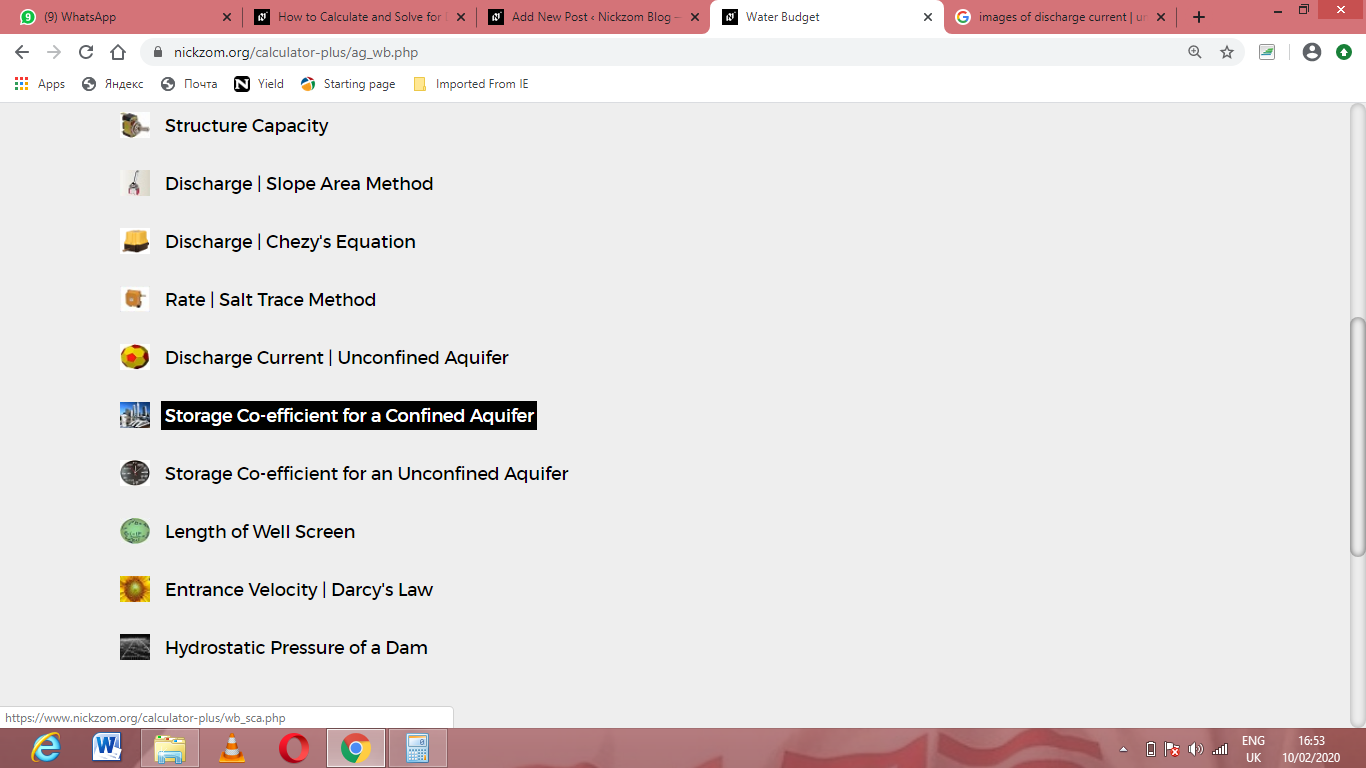The screenshot below displays the page or activity to enter your values, to get the answer for the storage co-efficient for a confined aquifer according to the respective parameters which are the Unit Weight of Water (γw), Confined Aquifer Thickness (H), Compressibility of the Aquifer Material (α), Porosity of Aquifer Material (n) and Compressibility of Water (β).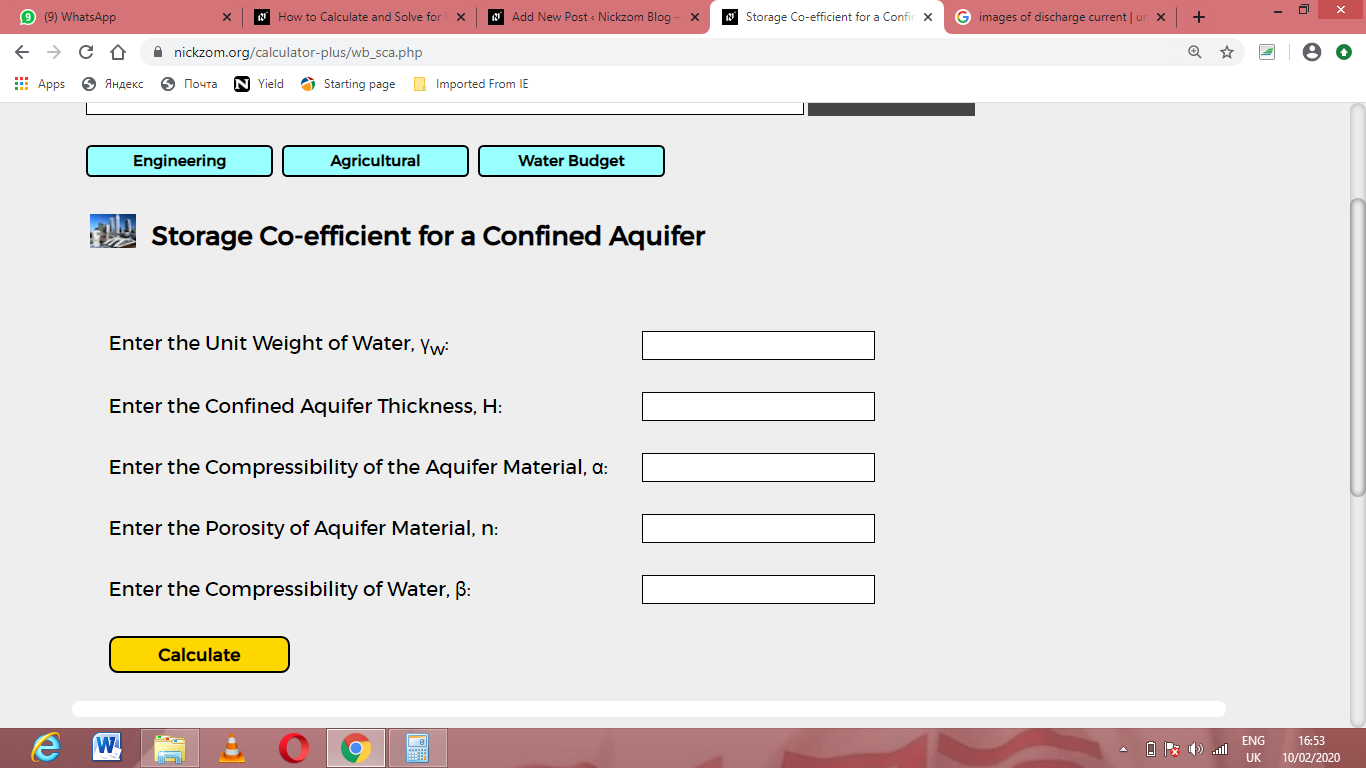Now, enter the values appropriately and accordingly for the parameters as required by the Unit Weight of Water (γw) is 8, Confined Aquifer Thickness (H) is 12, Compressibility of the Aquifer Material (α) is 21, Porosity of Aquifer Material (n) is 18 and Compressibility of Water (β) is 14.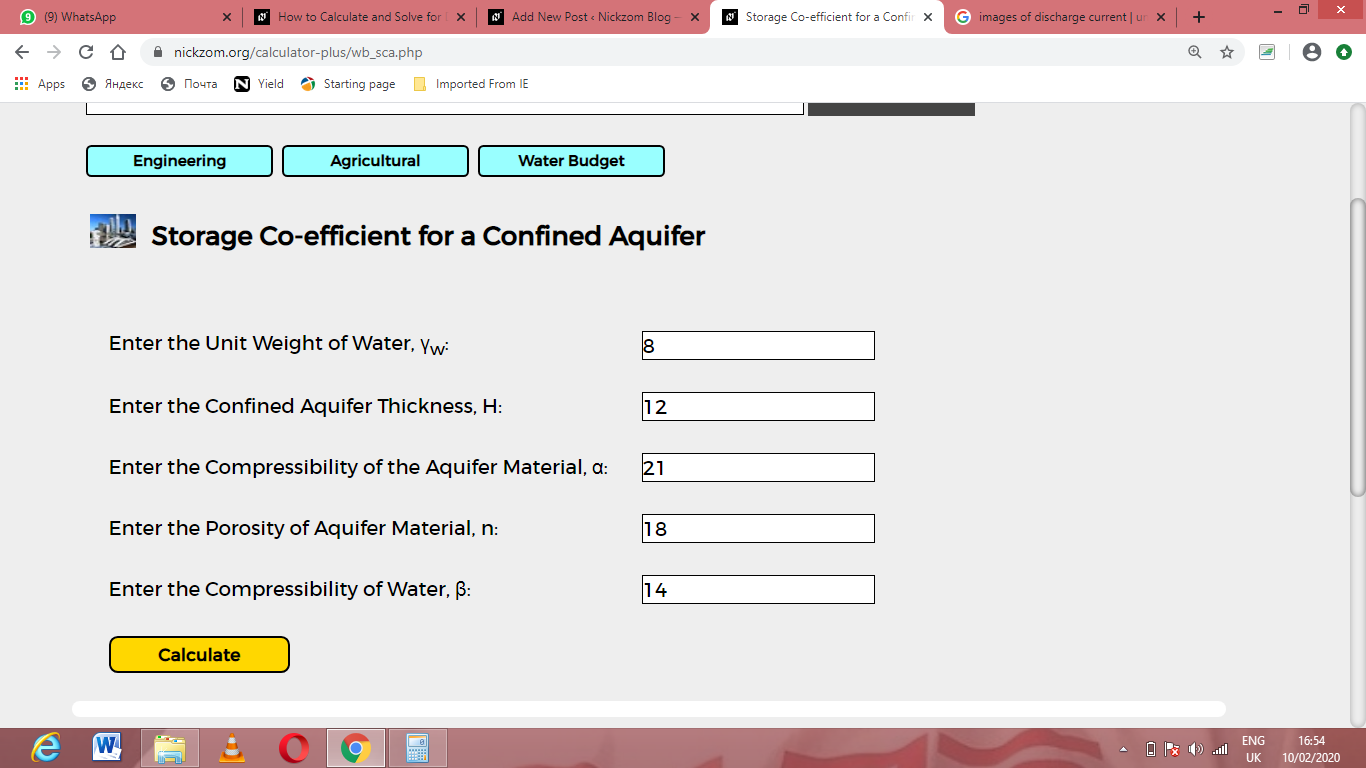Finally, Click on Calculate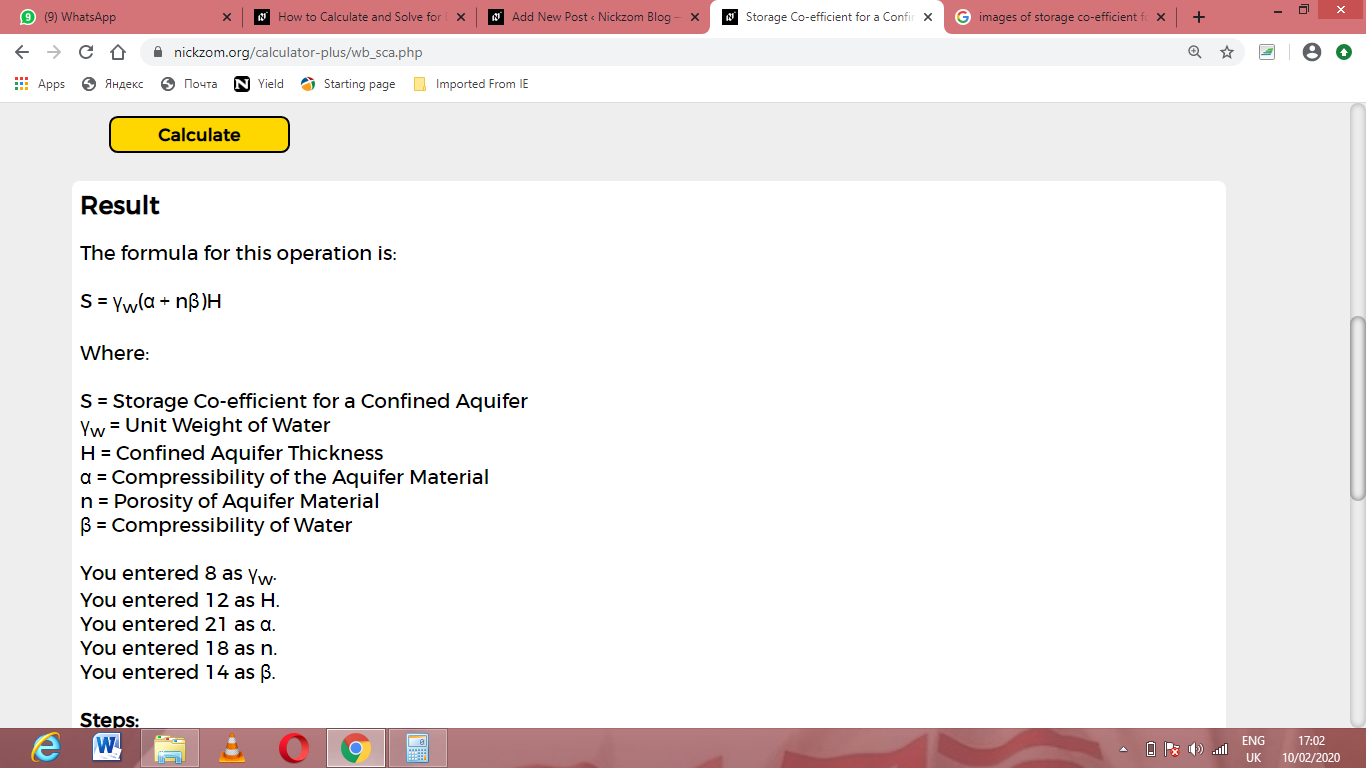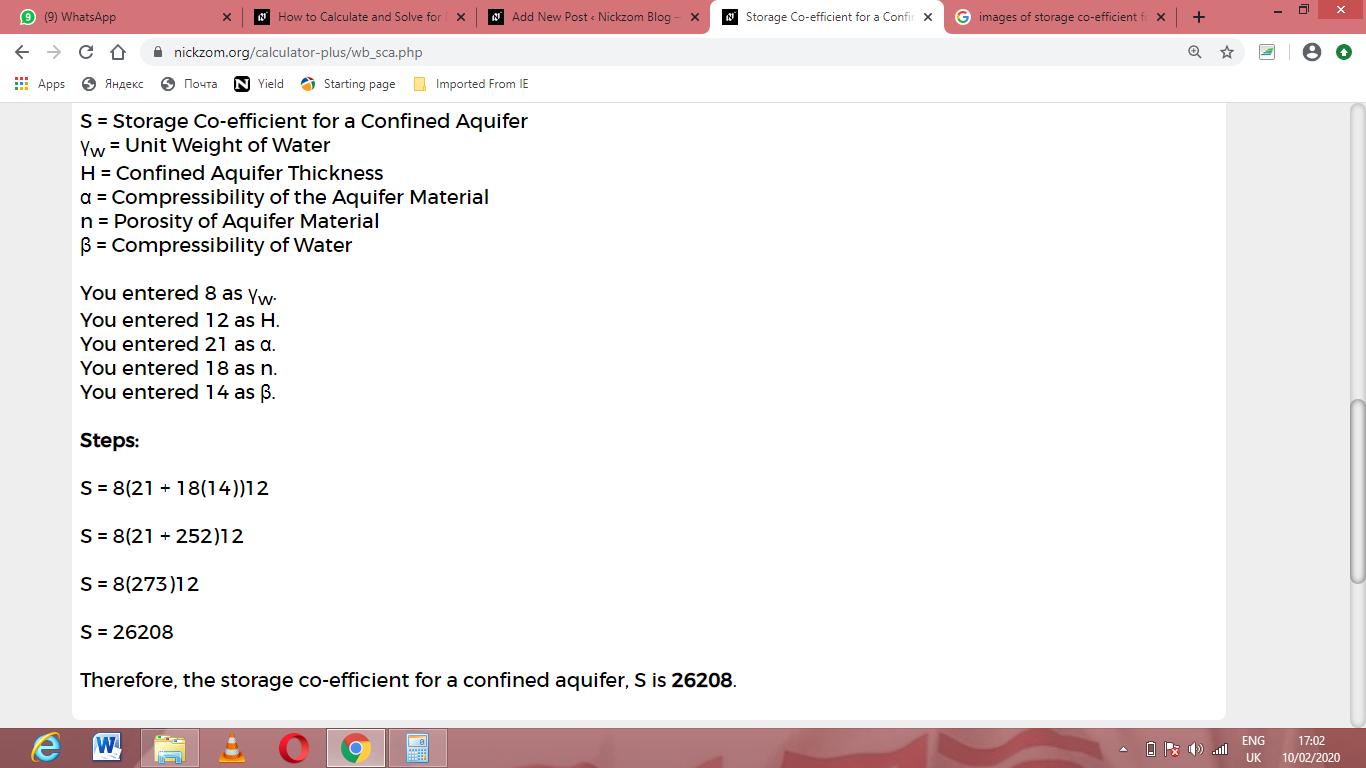As you can see from the screenshot above, Nickzom Calculator– The Calculator Encyclopedia solves for the storage co-efficient for a confined aquifer and presents the formula, workings and steps too.